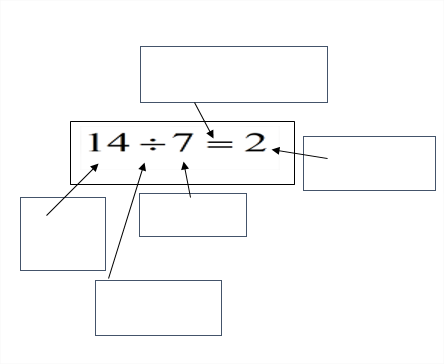# Division Of Integers Worksheet  for Class 7

In this worksheet, you will see step-by-step guidelines for the division of integers. It will help you in finding division answers after learning new concepts

## What is the “Division Of Integers Worksheet for Class 7”?

Division of Integers Class 7 Worksheet is a math worksheet made to assist learners to trigger their knowledge and understanding of concepts through math activities, word problems, assessment, and math worksheets. It will help them to create their practice questions and solve them.

## What is Division?

It’s one of four operations of arithmetic. It’s an operation opposite to multiplication.

The division is carried out by splitting a specific amount into equal parts.

### How will the “Division Of Integers Worksheet for Class 7 ” help you?

It will help you by providing step by step method for dividing integers. It makes you able to get the right solutions.

### Instructions on how to use the “Division Of Integers Worksheet for Class 7 ”

Use this worksheet to carefully analyze the concept behind division. Five practiced questions are provided after the lesson in order to exercise the learned concept.

A word problem is also designed to practice this division method in a new way.

At the end of this worksheet, a reflective section is provided in order to help the learner think about their own thinking and evaluate how they performed in the lesson.

Lastly, it also inquires the learner to come up with his/her own method of dividing fractions by whole numbers

## Conclusion

After understanding all the steps of division clearly, you will be able to solve or create your own practice questions. It will show you a relationship of integer numbers.

## Division Of Integers

Before anything else, let’s review some terms

Divisor: Number by which another number is to be divided

Dividend: Number that is being divided.

Quotient: The number resulting from the division of one number by another.

Whole Numbers: Numbers include natural numbers and zero.

Integers: Whole numbers (not fractional numbers) that can be positive, negative, or zero.

## Dividing Integers Step by Step

Step 1:

Write the Question first.

Step 2:
Divide them as a whole number first. Then put the suitable sign before the quotient.

## Dividing Whole Numbers By Fractions Worksheet

Identification:
Write the name of the terms labeled in the math equation below.Applying:
Solve the following

Problem Solving:

A baker is making pizza. He has 30 pounds of dough. Each pizza uses  4 pounds of dough. How many can he make?

Show your calculation in the box below.